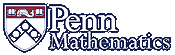Math 312: Linear Algebra: SyllabusWe will cover the following topics, although perhaps not in this order:

• systems of linear equations, existence and uniqueness, Gauss--Jordan elimination
• linear transformations and their geometry
• vector spaces and subspaces, dimension
• determinants and geometry
• eigenvectors and eigenvalues, diagonalization, complex eigenvalues
• dynamical systems, Markov chains